# What is vector shape

## Vectors¶

### Multiplication of vectors¶

Vectors can be multiplied either with a real number (a so-called “scalar”) or with other vectors.

Multiplication of a vector by a real number

Multiply a vector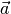with a real number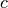, the result is a vector that has the same direction and the same sense of direction, but its magnitude by the factoris changed.

• Is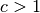, the vector is stretched.
• Is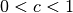, so the vector is compressed.
• Is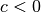, in addition to stretching or compressing the vector, the sense of direction is reversed.

This form of vector multiplication is often also called “S multiplication”.

A vector can be calculated arithmeticallywith a real numbermultiply by multiplying each component of the vector by this number:

Multiply a vectorwith the numberso it remains unchanged; so it always applies:

Multiply a vectorhowever, with the reciprocal of its amount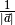, so you get the associated, on a unit of length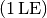normalized vector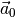:

In addition, the associative and distributive laws apply to the multiplication of scalars by vectors:

(10)¶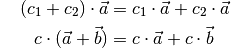The scalar product

The scalar product of two vectorsandis defined as the product of their amounts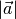and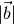, multiplied by the cosine of the angle between them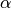:

(11)¶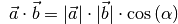If you write the two vectorsandin column form, the scalar product can be calculated component by component using the following formula:

(12)¶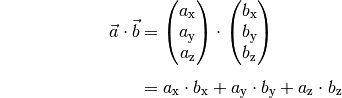The result is a scalar value, i.e. a number. The importance of the scalar product quickly becomes clear when one looks at a few special cases:

• The two vectors standandperpendicular to each other so is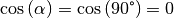. In this case, the scalar product results in the value zero: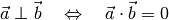With the help of this relationship it can be easily checked on the one hand, if two vectorsandstand perpendicular to each other. On the other hand, with a vectorwith only two given components using the component-wise representation the third component can be determined so that the vector is on the second vectorstands vertically.

Example:

The third component of the vector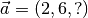shall be determined so that it is on the vectorstands vertically. So the following must apply: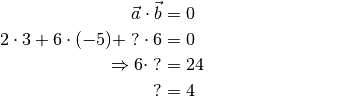Is the component you are looking for the same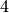, then both vectors are perpendicular to each other.

• The two vectors standandparallel to each other so is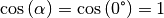. In this case, the scalar product is equal to the product of the amounts of both vectors.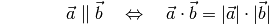This relationship has already been implicitly used to determine the amount of a certain vectorto calculate. You bet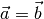, then: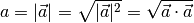The amountof the vector can thus be determined by taking the scalar product of the vector and taking the square root of the result. If you write the above equation component-wise, you get the usual absolute value equation (2).

• For any anglelets the product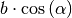geometrically as the “projection” of the vector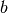on the vector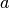interpret. The projection clearly corresponds to the “shadow cast” of the vectorthat is perpendicular to aincident lighting.

The value of the scalar product is therefore generally equal to the magnitude of the first vector, multiplied by the perpendicular projection of the second vector onto the first.

Since the scalar product is easy to calculate component by component, it can also be used to calculate the angle between two vectors or a vector and one of the axes of a (Cartesian) coordinate system. For the angle between two vectors, based on equation (11), the following applies: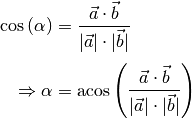In order to calculate the angle, one only has to calculate the scalar product and divide it by the product of the two vector amounts; the arc cosine of this value gives the angle you are looking for.

In order to calculate the angle between a vector and the individual spatial axes, this can also be calculated using vectors of lengthand can be represented with only a single vector component, for example the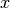Axis through the vector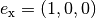. This gives: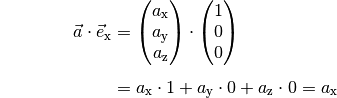The same applies to the scalar products ofwith the other two spatial axes. The general formula (11) of the scalar product can thus be used to search for the angleto be resolved: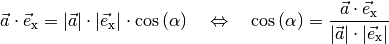If you set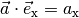and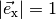into the above equation, it follows: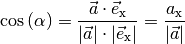For the angles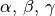betweenand the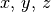-Axes is therefore:

(13)¶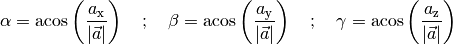The vector product

The vector product of two vectorsandresults in a vector that is perpendicular to each of the two vectors and. This definition only makes sense from a three-dimensional space.

The magnitude of the vector product of two vectorsandis equal to the product of their amountsand, multiplied by the sine of the angle between them:

(14)¶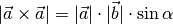If you write the two vectorsandin column form, the vector product can be calculated component by component using the following formula:

(15)¶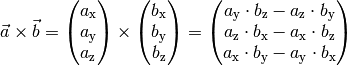The vector product is used in analytical geometry and in engineering. For example, for two given direction vectors that describe a plane, a third “norm vector” can be found with the help of the vector product, which is perpendicular to the plane. In physics, for example, the vector product is used to calculate torques and angular momentum.

Remarks: